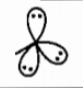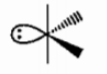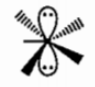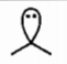In which of the following molecular shape ${d}_{{z}^{2-}}$ orbital must not be involved in bonding?

(a) Pentagonal planar

(b) Trigonal planar

(c) Linear

(d) Square planar

Concept Questions :-

Hybridisation
High Yielding Test Series + Question Bank - NEET 2020

Difficulty Level:

Consider the following reaction:

${\mathrm{MX}}_{4}+\mathrm{X}{\text{'}}_{2}\to {\mathrm{MX}}_{4}\mathrm{X}{\text{'}}_{2}$

If atomic number of M is 52 and X and X' are halogens and X' is more electronegative than X.

Then choose correct statement regarding given information:

(a) Both X' atoms occupy axial positions which are formed by overlapping of p and d-orbitals only

(b) All

(c) Central atom 'M' does not use anyone valence non-axial set of d-orbital in hybridization of final product

(d) Hybridization of central atom 'M' remains same in both reactant and final product.

Concept Questions :-

Hybridisation
High Yielding Test Series + Question Bank - NEET 2020

Difficulty Level:

Which atom can have more than eight valence electrons when it is forming covalent bond?

(a) H

(b) N

(c) F

(d) Cl

Concept Questions :-

Types of Bonding
High Yielding Test Series + Question Bank - NEET 2020

Difficulty Level:

Select the incorrect match:

Statement                               Shape                                    Example

(a) Bond pair has > 75%HF

p-character

(b) Reduction in axial bond${\mathrm{SF}}_{4}$

is more than that of in

equatorial bond angle

(c) Two axial d-orbitals and${\mathrm{XeF}}_{5}^{-}$

one non-axial d-orbitals are

used in hybridization

(d) Two p-orbitals are used${\mathrm{SnCl}}_{2}$

in hybridization

Concept Questions :-

Hybridisation
High Yielding Test Series + Question Bank - NEET 2020

Difficulty Level:

Which bond is expected to be the least polar?

1. O-F

2. P-F

3. Si-N

4. B-F

Concept Questions :-

Polarity
High Yielding Test Series + Question Bank - NEET 2020

Difficulty Level:

Select correct statement(s) :

(a) Acidic strength of $\mathrm{HBr}>\mathrm{HCl}$ but reverse is true for their reducing property

(b) Basic strength of ${\mathrm{PH}}_{3}>{\mathrm{AsH}}_{3}$ but reverse is true for their bond angle

(c) Dipole moment of ${\mathrm{CH}}_{3}\mathrm{Cl}>{\mathrm{CH}}_{3}\mathrm{F}$ but reverse is true for their $\mathrm{H}\stackrel{^}{\mathrm{C}}\mathrm{H}$ bond angle

(d) ${\mathrm{K}}_{{\mathrm{a}}_{1}}$ of fumaric acid is higher than maleic acid but reverse is true for their ${\mathrm{K}}_{{\mathrm{a}}_{2}}$

Concept Questions :-

Acid, Base and Fajan's Rule
High Yielding Test Series + Question Bank - NEET 2020

Difficulty Level:

Which set contains only covalently bonded molecules?

(a)

(b)

(c)

(d)

Concept Questions :-

Covalent Bonding
High Yielding Test Series + Question Bank - NEET 2020

Difficulty Level:

In which of the following species, d-orbitals having xz and yz two nodal planes involved in hybridization of central atom?

(a) ${\mathrm{IO}}_{2}{\mathrm{F}}_{2}^{-}$                                  (b) ${\mathrm{ClF}}_{4}^{-}$

(c) ${\mathrm{IF}}_{7}$                                       (d) None of these

Concept Questions :-

Hybridisation
High Yielding Test Series + Question Bank - NEET 2020

Difficulty Level:

Select pair of compound in which both have differant hybridization but have same molecular geomatery:

(a)  $B{F}_{3,}Br{F}_{3}$

(b) $IC{I}_{2},BeC{I}_{2}$

(c)$BC{I}_{3,}PC{I}_{3}$

(d) $PC{I}_{3,}NC{I}_{3}$

Concept Questions :-

Hybridisation
High Yielding Test Series + Question Bank - NEET 2020

Difficulty Level:

Which molecule does not exist?

(a) $O{F}_{2}$

(b) $O{F}_{4}$

(c) $S{F}_{2}$

(d) $S{F}_{4}$

Concept Questions :-

Covalent Bonding